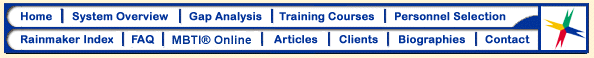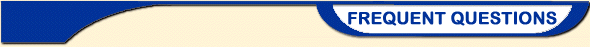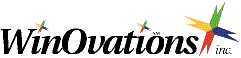Related Web Sites Upcoming Events and Conferences Search the WinOvations Web Site

#Questions...?### I only have the scores for all of my 8 individual preferences from the MBTI® instrument, but not an overall set of 4 numerical scores. How do I convert them to four overall preference scores?

Your MBTI® instrument facilitator should have provided you with the four overall scores. You could ask them to do so, or calculate them yourself.

Example: Suppose your individual scores were as follows:

E=22, I=2 (This is Extroversion-Introversion preference scale)
S=24, N=10 (This is the Sensory-iNtuitive preference scale)
T=8, F=15 (This is the Thinking-Feeling Preference scale)
J=20, P=8 (This is the Judging-Perceiving preference scale)

Convert these scores to an overall preference for each of the four scales as follows:
a. Subtract the smaller score from the larger score, and multiply by 2.
b. If the larger score is the first of each of the pairs above, subtract 1 point.
If the larger score is the second of each of the pairs above, add 1 point.
(Ref 1, 2)

Example using the numbers above:

E minus I=22-2=20. 20x2=40. 40-1=39. Overall preference and score: "E39"
S minus N=24-10=14. 14x2=28. 28-1=27. Overall preference and score: "S27"
F minus T=15-8=7. 7x2=14. 14+1=15. Overall preference and score: "F15"
J minus P=20-8=12. 12x2=24. 24-1=23. Overall preference and score: "J23"

Hence, this individual would have a preference of: E39; S27; F15; J23. These are the scores that are used to calculate the Creativity Index.### #2. Question:

I took the MBTI® instrument years ago, and do not remember where the results are, but do remember my overall preferences and their approximate strengths. Can I use these results to estimate my Creativity Index?

You really should retake the instrument, and get numerical preference scores.

However, if it is impractical to do this, you can estimate your preference scores. The possible range for each of the overall preference scores is shown below. From these, you can "guesstimate" approximate numerical values for the preferences.

E: Extroversion range of preferences = 1 to 51
I: Introversion range of preferences = 1 to 57

S: Sensory range of preferences = 1 to 67
N: iNtutition range of preferences = 1 to 51

T: Thinking range of preferences = 1 to 65 for males & females
F: Feeling range of preferences = 1 to 39 for males, 1 to 43 for females

J: Judging range of preferences = 1 to 55
P: Perceiving range of preferences = 1 to 61

(Ref 1, 2)

Example: say you recall that most of your preferences were ESFJ, and that they were all reasonably definite, but not extremely so, except that E was close to I. You might then estimate that your MBTI® instrument preferences and numbers are very roughly:

E 10; S 30; T 30 and J 27.

##### These numbers can be used to estimate your Creativity Index, but it is better to have taken the MBTI® instrument directly.### #3. Question:

The results from the instrument did not provide my "true type", which I realized after some discussion with a trained MBTI® instrument facilitator. For example, the instrument indicated that I was an INTP, but after discussing it and reading several of the 16 MBTI® instrument type descriptions, I decided I am really an INFP. What should I do in using the scores from the instrument to estimate my Creativity Index?

This is a relatively common occurrence. What we recommend is that you use the numerical scores for the scales that you are confident of, and use an estimation for the one (or more) scores where your "true type" is different from the indicated by the instrument.

Example: Say that you are confident of the preference scores from the instrument for I, N and P. Use these preference scores directly in the calculation of the Creativity Index.

However, you believe that "F" fits you better that "T". Depending on how strong you think this preference really is, choose a numerical score for "F", as described in question #2 above. If it is a relatively weak preference, choose a low number (1-10). Typically this kind of switch happens where a preference is not particularly strong. Perhaps the score for "T" was only 5. It might then be appropriate to use a relatively low score for "F" of around 5. Both of these scores are close to the "middle."

Note that only the individual can estimate what this score should be, and it is possible to have a very strong "true-type preference" that is opposite of what the instrument provided.

Note: If you have not taken the MBTI® instrument, you can arrange to do so through a qualified MBTI® instrument administrator. Greg Stevens at WinOvations, Inc. is a one such administrator.### #4 Question:

How do I convert typical MBTI® instrument scores into continuous scores, for use in the Creativity Index, and the Rainmaker Index?  (Ref 1, 2)

Note: You can use our Calculator page to automatically do the following calculations.

In the following example, the person is supplied only with the raw MBTI® Form G subscores for each of the 4 indices measured by MBTI® Form G, as follows:

E=3

I=21

S=5

N=24

T=23

F=6

J=6

P=21

Most people will not need to do this first step. Your MBTI® instrument facilitator really should have provided you with an overall score for each of the four axes. Most do. However, in this example it was not done, so we need to first calculate the overall MBTI® instrument scores.

The overall MBTI® instrument scores are calculated by subtracting the smaller score from the larger one, multiplying by two, and then either adding or subtracting one. You subtract "1" if the larger of the two are E, S, T or J. You add "1" to the score if the larger is I, N, F or P. For example, the overall MBTI® instrument scores for each of the four axes are shown below. This is the format that MBTI® instrument scores are supposed to be reported in:

I-37 (Example calculation: I21-E3 = 18, 18 x 2 = 36, 36 + 1 = 37)

N-39 (Example calculation: N24-S5 = 19, 19 x 2 = 38, 38 + 1 = 39)

T-33 (Example calculation: T23-F6 = 17, 17 x 2 = 34, 34 - 1 = 33)

P-31 (Example calculation: P21-J6 = 15, 15 x 2 = 30, 30 + 1 = 31)

The next step is to calculate MBTI® instrument continuous scores. The scores typically reported directly above have zero as the midpoint for each of the four scales. In other words, for the S to N (Sensory to iNtuitive) scale there is a continuum going from S to N, with zero in the midpoint.

To use statistics with the MBTI® instrument scores (to see correlations between the strength of the MBTI® instrument preference on individual performance), we need to first convert these scores to continuous scores with 100 at the midpoints, instead of zero. Continuous MBTI® instrument scores are not normally reported to participants, so everyone interested in calculating their Creativity Index or their Rainmaker Index has to first convert their overall MBTI® instrument scores to Continuous MBTI® instrument scores. Continuous MBTI® instrument scores are calculated from the overall MBTI® instrument scores as follows:

For overall preferences of E, S, T or J we subtract the overall MBTI® instrument score from 100.

For overall preferences of I, N, F or P we add the overall score to 100.

Example: Using the above overall MBTI® instrument preference scores for INTP, calculate the continuous MBTI® instrument scores, which are subsequently used to calculate the Creativity Index, and the Rainmaker Index:

EI continuous score = 137 (100 + I score of 37)

SN continuous score = 139 (100 + N score of 39)

TF continuous score = 67 (100 - T score of 33)

JP continuous score = 131 (100 + P score of 31)

Creativity Index:

The Creativity Index uses the above continuous scores. The formula for the CI is as follows:

CI = 3 x (SN) + (JP) - (EI) - 0.5 x (TF)    (Ref 3)

Example: using the same INTP participant

CI = 3 x (139) + (131) - (137) - 0.5 x (67)

= 417 + 131 - 137 - 33.5

= 377.5 This is a relatively high Creativity Index

The range of scores possible with the Creativity Index is from negative 84.5 to positive 547.5. The mean CI in the very large CAPT database is 235. The mean in our study of 69 business analysts was 285, and the median was 273. Harrison Gough, who developed the CI, found that CI’s above 350 correlate with people who are highly creative in many different fields. We have found that analysts in the top third of the CI out-earn those in the bottom third by a factor of 11 times.

Rainmaker Index:

The Rainmaker Index, which correlates with profits that result from the "fuzzy front end" of new product development (provided that the analysts are first trained and extensively coached in the WinOvations system of new business development), is also calculated from MBTI® instrument continuous scores.

The formula for the Rainmaker Index from our paper is as follows:

Rainmaker Index = (SN) - (TF)

Example:

Rainmaker Index = 139 - 67

= 72 (This is quite high.)

The possible range for the Rainmaker Index is from negative 110 to positive 116. The mean in the sample we studied was 27.8, and the median was 25. We have found that Rainmaker Index’s above 46 are quite high. Analysts with Rainmaker Indexes above 46 (i.e. in the top third of the group) identified the great majority of profits, outearning those with Rainmaker Indices in the bottom third by a factor of 95 times.

#####Myers-Briggs Type Indicator and MBTI are registered trademarks of Consulting Psychologists Press, Inc.

References:

1. Margaret Hartzler, and Katherine Myers, Linda Berens, Stephanie Rogers. 1992 The MBTI® Professional Qualifying Program Notebook, "Introduction to Theory" Gaitnersburg, MD, Type Resources, Inc. p8.

2. The MBTI® Form G Scoring Template, Copyright 1977 by Isabel Briggs Myers, Published by Consulting Psychology Press Inc, Palo Alto, CA.

3. Gough, Harrison G. 1979. "A creative personality scale for the adjective checklist." Journal of Personality and Social Psychology p37 1398-1405

#####A Six Sigma Breakthrough in Innovation

 |  Home  |  System Overview  |  Gap Analysis  |  Training Courses  |  Personnel Selection  | |  Rainmaker Index  |  FAQ  |  MBTI® Online  |  Articles  |  Clients  |  Biographies  |  Contact  | Search the WinOvations Web Site